Q-Chem 4.3 User’s Manual

# 4.2 Hartree–Fock Calculations

## 4.2.1 The Hartree-Fock Equations

As with much of the theory underlying modern quantum chemistry, the Hartree-Fock approximation was developed shortly after publication of the Schrödinger equation, but remained a qualitative theory until the advent of the computer. Although the HF approximation tends to yield qualitative chemical accuracy, rather than quantitative information, and is generally inferior to many of the DFT approaches available, it remains as a useful tool in the quantum chemist’s toolkit. In particular, for organic chemistry, HF predictions of molecular structure are very useful.

Consider once more the Roothaan-Hall equations, Eq. (4.11), or the Pople-Nesbet equations, Eq. (4.13), which can be traced back to the integro–differential Eq. (4.8) in which the effective potential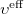depends on the SCF methodology. In a restricted HF (RHF) formalism, the effective potential can be written as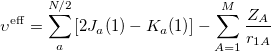(4.14)

where the Coulomb and exchange operators are defined as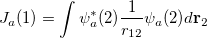(4.15)

and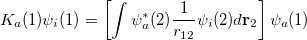(4.16)

respectively. By introducing an atomic orbital basis, we obtain Fock matrix elements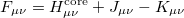(4.17)

where the core Hamiltonian matrix elements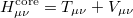(4.18)

consist of kinetic energy elements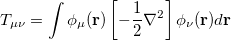(4.19)

and nuclear attraction elements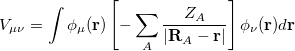(4.20)

The Coulomb and Exchange elements are given by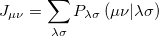(4.21)

and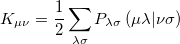(4.22)

respectively, where the density matrix elements are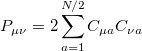(4.23)

and the two electron integrals are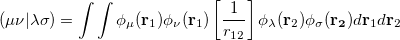(4.24)

Note: The formation and utilization of two-electron integrals is a topic central to the overall performance of SCF methodologies. The performance of the SCF methods in new quantum chemistry software programs can be quickly estimated simply by considering the quality of their atomic orbital integrals packages. See Appendix B for details of Q-Chem’s AOINTS package.

Substituting the matrix element in Eq. (4.17) back into the Roothaan-Hall equations, Eq. (4.11), and iterating until self-consistency is achieved will yield the Restricted Hartree-Fock (RHF) energy and wavefunction. Alternatively, one could have adopted the unrestricted form of the wavefunction by defining an alpha and beta density matrix: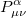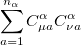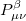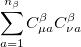(4.25)

The total electron density matrix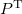is simply the sum of the alpha and beta density matrices. The unrestricted alpha Fock matrix,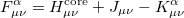(4.26)

differs from the restricted one only in the exchange contributions where the alpha exchange matrix elements are given by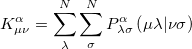(4.27)

## 4.2.2 Wavefunction Stability Analysis

At convergence, the SCF energy will be at a stationary point with respect to changes in the MO coefficients. However, this stationary point is not guaranteed to be an energy minimum, and in cases where it is not, the wavefunction is said to be unstable. Even if the wavefunction is at a minimum, this minimum may be an artifact of the constraints placed on the form of the wavefunction. For example, an unrestricted calculation will usually give a lower energy than the corresponding restricted calculation, and this can give rise to a RHF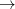UHF instability.

To understand what instabilities can occur, it is useful to consider the most general form possible for the spin orbitals: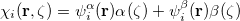(4.28)

Here, the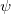’s are complex functions of the Cartesian coordinates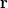, andand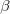are spin eigenfunctions of the spin-variable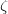. The first constraint that is almost universally applied is to assume the spin orbitals depend only on one or other of the spin-functionsor. Thus, the spin-functions take the form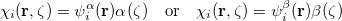(4.29)

where the’s are still complex functions. Most SCF packages, including Q-Chem’s, deal only with real functions, and this places an additional constraint on the form of the wavefunction. If there exists a complex solution to the SCF equations that has a lower energy, the wavefunction will exhibit either a RHFCRHF or a UHFCUHF instability. The final constraint that is commonly placed on the spin-functions is that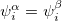, i.e., the spatial parts of the spin-up and spin-down orbitals are the same. This gives the familiar restricted formalism and can lead to a RHFUHF instability as mentioned above. Further details about the possible instabilities can be found in Ref. Seeger:1977.

Wavefunction instabilities can arise for several reasons, but frequently occur if

• There exists a singlet diradical at a lower energy then the closed-shell singlet state.

• There exists a triplet state at a lower energy than the lowest singlet state.

• There are multiple solutions to the SCF equations, and the calculation has not found the lowest energy solution.

If a wavefunction exhibits an instability, the seriousness of it can be judged from the magnitude of the negative eigenvalues of the stability matrices. These matrices and eigenvalues are computed by Q-Chem’s Stability Analysis package, which was implemented by Dr Yihan Shao. The package is invoked by setting the STABILITY_ANALYSIS $rem variable is set to TRUE. In order to compute these stability matrices Q-Chem must first perform a CIS calculation. This will be performed automatically, and does not require any further input from the user. By default Q-Chem computes only the lowest eigenvalue of the stability matrix. This is usually sufficient to determine if there is a negative eigenvalue, and therefore an instability. Users wishing to calculate additional eigenvalues can do so by setting the CIS_N_ROOTS$rem variable to a number larger than 1.

Q-Chem’s Stability Analysis package also seeks to correct internal instabilities (RHFRHF or UHFUHF). Then, if such an instability is detected, Q-Chem automatically performs a unitary transformation of the molecular orbitals following the directions of the lowest eigenvector, and writes a new set of MOs to disk. One can read in these MOs as an initial guess in a second SCF calculation (set the SCF_GUESS $rem variable to READ), it might also be desirable to set the SCF_ALGORITHM to GDM. In cases where the lowest-energy SCF solution breaks the molecular point-group symmetry, the SYM_IGNORE$rem should be set to TRUE.

Note: The stability analysis package can be used to analyze both DFT and HF wavefunctions.

## 4.2.3 Basic Hartree-Fock Job Control

In brief, Q-Chem supports the three main variants of the Hartree-Fock method. They are:

• Restricted Hartree-Fock (RHF) for closed shell molecules. It is typically appropriate for closed shell molecules at their equilibrium geometry, where electrons occupy orbitals in pairs.

• Unrestricted Hartree-Fock (UHF) for open shell molecules. Appropriate for radicals with an odd number of electrons, and also for molecules with even numbers of electrons where not all electrons are paired (for example stretched bonds and diradicaloids).

• Restricted open shell Hartree-Fock (ROHF) for open shell molecules, where the alpha and beta orbitals are constrained to be identical.

Only two $rem variables are required in order to run Hartree-Fock (HF) calculations: • METHOD must be set to HF. • A valid keyword for BASIS must be specified (see Chapter 7). In slightly more detail, here is a list of basic$rem variables associated with running Hartree-Fock calculations. See Chapter 7 for further detail on basis sets available and Chapter 8 for specifying effective core potentials.

JOBTYPE
 Specifies the type of calculation.

TYPE:
 STRING

DEFAULT:
 SP

OPTIONS:
 SP Single point energy. OPT Geometry Minimization. TS Transition Structure Search. FREQ Frequency Calculation. FORCE Analytical Force calculation. RPATH Intrinsic Reaction Coordinate calculation. NMR NMR chemical shift calculation. ISSC Indirect nuclear spin–spin coupling calculation. BSSE BSSE calculation. EDA Energy decomposition analysis.

RECOMMENDATION:
 Job dependent

METHOD
 Specifies the level of theory.

TYPE:
 STRING

DEFAULT:
 No default

OPTIONS:
 HF Exact (Hartree-Fock).

RECOMMENDATION:
 Use HF for Hartree-Fock calculations.

BASIS
 Specifies the basis sets to be used.

TYPE:
 STRING

DEFAULT:
 No default basis set

OPTIONS:
 General, Gen User defined ($basis keyword required). Symbol Use standard basis sets as per Chapter 7. Mixed Use a mixture of basis sets (see Chapter 7). RECOMMENDATION:  Consult literature and reviews to aid your selection. PRINT_ORBITALS  Prints orbital coefficients with atom labels in analysis part of output. TYPE:  INTEGER/LOGICAL DEFAULT:  FALSE OPTIONS:  FALSE Do not print any orbitals. TRUE Prints occupied orbitals plus 5 virtuals. NVIRT Number of virtuals to print. RECOMMENDATION:  Use TRUE unless more virtuals are desired. THRESH  Cutoff for neglect of two electron integrals.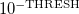(THRESH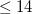). TYPE:  INTEGER DEFAULT:  8 For single point energies. 10 For optimizations and frequency calculations. 14 For coupled-cluster calculations. OPTIONS:for a threshold of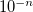. RECOMMENDATION:  Should be at least three greater than SCF_CONVERGENCE. Increase for more significant figures, at greater computational cost. SCF_CONVERGENCE  SCF is considered converged when the wavefunction error is less that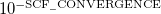. Adjust the value of THRESH at the same time. Note that in Q-Chem 3.0 the DIIS error is measured by the maximum error rather than the RMS error as in previous versions. TYPE:  INTEGER DEFAULT:  5 For single point energy calculations. 8 For geometry optimizations and vibrational analysis. 8 For SSG calculations, see Chapter 5. OPTIONS:  User-defined RECOMMENDATION:  Tighter criteria for geometry optimization and vibration analysis. Larger values provide more significant figures, at greater computational cost. UNRESTRICTED  Controls the use of restricted or unrestricted orbitals. TYPE:  LOGICAL DEFAULT:  FALSE (Restricted) Closed-shell systems. TRUE (Unrestricted) Open-shell systems. OPTIONS:  TRUE (Unrestricted) Open-shell systems. FALSE Restricted open-shell HF (ROHF). RECOMMENDATION:  Use default unless ROHF is desired. Note that for unrestricted calculations on systems with an even number of electrons it is usually necessary to break alpha/beta symmetry in the initial guess, by using SCF_GUESS_MIX or providing$occupied information (see Section 4.4 on initial guesses).

Listed below are a number of useful options to customize a Hartree-Fock calculation. This is only a short summary of the function of these $rem variables. A full list of all SCF-related variables is provided in Appendix C. A number of other specialized topics (large molecules, customizing initial guesses, and converging the calculation) are discussed separately in Sections 4.6, 4.4, and 4.5, respectively. INTEGRALS_BUFFER  Controls the size of in-core integral storage buffer. TYPE:  INTEGER DEFAULT:  15 15 Megabytes. OPTIONS:  User defined size. RECOMMENDATION:  Use the default, or consult your systems administrator for hardware limits. DIRECT_SCF  Controls direct SCF. TYPE:  LOGICAL DEFAULT:  Determined by program. OPTIONS:  TRUE Forces direct SCF. FALSE Do not use direct SCF. RECOMMENDATION:  Use default; direct SCF switches off in-core integrals. METECO  Sets the threshold criteria for discarding shell-pairs. TYPE:  INTEGER DEFAULT:  2 Discard shell-pairs below. OPTIONS:  1 Discard shell-pairs four orders of magnitude below machine precision. 2 Discard shell-pairs below 10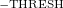. RECOMMENDATION:  Use default. STABILITY_ANALYSIS  Performs stability analysis for a HF or DFT solution. TYPE:  LOGICAL DEFAULT:  FALSE OPTIONS:  TRUE Perform stability analysis. FALSE Do not perform stability analysis. RECOMMENDATION:  Set to TRUE when a HF or DFT solution is suspected to be unstable. SCF_PRINT  Controls level of output from SCF procedure to Q-Chem output file. TYPE:  INTEGER DEFAULT:  0 Minimal, concise, useful and necessary output. OPTIONS:  0 Minimal, concise, useful and necessary output. 1 Level 0 plus component breakdown of SCF electronic energy. 2 Level 1 plus density, Fock and MO matrices on each cycle. 3 Level 2 plus two-electron Fock matrix components (Coulomb, HF exchange and DFT exchange-correlation matrices) on each cycle. RECOMMENDATION:  Proceed with care; can result in extremely large output files at level 2 or higher. These levels are primarily for program debugging. SCF_FINAL_PRINT  Controls level of output from SCF procedure to Q-Chem output file at the end of the SCF. TYPE:  INTEGER DEFAULT:  0 No extra print out. OPTIONS:  0 No extra print out. 1 Orbital energies and break-down of SCF energy. 2 Level 1 plus MOs and density matrices. 3 Level 2 plus Fock and density matrices. RECOMMENDATION:  The break-down of energies is often useful (level 1). KS_GAP_PRINT  Control printing of (generalized Kohn-Sham) HOMO-LUMO gap information. TYPE:  Boolean DEFAULT:  false OPTIONS:  false (default) do not print gap information true print gap information RECOMMENDATION:  Use in conjunction with KS_GAP_UNIT if true. KS_GAP_UNIT  Unit for KS_GAP_PRINT and FOA_FUNDGAP TYPE:  INTEGER DEFAULT:  0 OPTIONS:  0 (default) hartrees 1 eV RECOMMENDATION:  none DIIS_SEPARATE_ERRVEC  Control optimization of DIIS error vector in unrestricted calculations. TYPE:  LOGICAL DEFAULT:  FALSE Use a combined alpha and beta error vector. OPTIONS:  FALSE Use a combined alpha and beta error vector. TRUE Use separate error vectors for the alpha and beta spaces. RECOMMENDATION:  When using DIIS in Q-Chem a convenient optimization for unrestricted calculations is to sum the alpha and beta error vectors into a single vector which is used for extrapolation. This is often extremely effective, but in some pathological systems with symmetry breaking, can lead to false solutions being detected, where the alpha and beta components of the error vector cancel exactly giving a zero DIIS error. While an extremely uncommon occurrence, if it is suspected, set DIIS_SEPARATE_ERRVEC to TRUE to check. ## 4.2.5 Examples Provided below are examples of Q-Chem input files to run ground state, Hartree-Fock single point energy calculations. Example 4.19 Example Q-Chem input for a single point energy calculation on water. Note that the declaration of the single point$rem variable and level of theory to treat correlation are redundant because they are the same as the Q-Chem defaults.

$molecule 0 1 O H1 O oh H2 O oh H1 hoh oh = 1.2 hoh = 120.0$end

$rem JOBTYPE sp Single Point energy METHOD hf Hartree-Fock BASIS sto-3g Basis set$end

$comment HF/STO-3G water single point calculation$end


Example 4.20  UHF/6-311G calculation on the Lithium atom. Note that correlation and the job type were not indicated because Q-Chem defaults automatically to no correlation and single point energies. Note also that, since the number of alpha and beta electron differ, MOs default to an unrestricted formalism.

$molecule 0,2 3$end

$rem METHOD HF Hartree-Fock BASIS 6-311G Basis set$end


Example 4.21  ROHF/6-311G calculation on the Lithium atom. Note again that correlation and the job type need not be indicated.

$molecule 0,2 3$end

$rem METHOD hf Hartree-Fock UNRESTRICTED false Restricted MOs BASIS 6-311G Basis set$end


Example 4.22  RHF/6-31G stability analysis calculation on the singlet state of the oxygen molecule. The wavefunction is RHFUHF unstable.

$molecule 0 1 O O 1 1.165$end

$rem METHOD hf Hartree-Fock UNRESTRICTED false Restricted MOs BASIS 6-31G(d) Basis set STABILITY_ANALYSIS true Perform a stability analysis$end


## 4.2.6 Symmetry

Symmetry is a powerful branch of mathematics and is often exploited in quantum chemistry, both to reduce the computational workload and to classify the final results obtained [18, 19, 20]. Q-Chem is able to determine the point group symmetry of the molecular nuclei and, on completion of the SCF procedure, classify the symmetry of molecular orbitals, and provide symmetry decomposition of kinetic and nuclear attraction energy (see Chapter 10).

Molecular systems possessing point group symmetry offer the possibility of large savings of computational time, by avoiding calculations of integrals which are equivalent i.e., those integrals which can be mapped on to one another under one of the symmetry operations of the molecular point group. The Q-Chem default is to use symmetry to reduce computational time, when possible.

There are several keywords that are related to symmetry, which causes frequent confusion. SYM_IGNORE controls symmetry throughout all modules. The default is FALSE. In some cases it may be desirable to turn off symmetry altogether, for example if you do not want Q-Chem to reorient the molecule into the standard nuclear orientation, or if you want to turn it off for finite difference calculations. If the SYM_IGNORE \$rem is set to TRUE then the coordinates will not be altered from the input, and the point group will be set to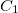.

The SYMMETRY (an alias for ISYM_RQ) keyword controls symmetry in some integral routines. It is set to FALSE by default. Note that setting it to FALSE does not turn point group symmetry off, and does not disable symmetry in the coupled-cluster suite (CCMAN and CCMAN2), which is controlled by CC_SYMMETRY (see Chapters 5 and 6), although we noticed that sometimes it may mess up the determination of orbital symmetries, possibly due to numeric noise. In some cases, SYMMETRY=TRUE can cause problems (poor convergence and crazy SCF energies) and turning it off can help.

Note: The user should be aware about different conventions for defining symmetry elements. The arbitrariness affects, for example,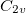point group. The specific choice affects how the irreps in the affected groups are labeled. For example,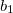and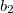irreps inare flipped when using different conventions. Q-Chem uses non-Mulliken symmetry convention. See http://iopenshell.usc.edu/howto/symmetry for detailed explanations.

SYMMETRY
 Controls the efficiency through the use of point group symmetry for calculating integrals.

TYPE:
 LOGICAL

DEFAULT:
 TRUE Use symmetry for computing integrals.

OPTIONS:
 TRUE Use symmetry when available. FALSE Do not use symmetry. This is always the case for RIMP2 jobs

RECOMMENDATION:
 Use default unless benchmarking. Note that symmetry usage is disabled for RIMP2, FFT, and QM/MM jobs.

SYM_IGNORE
 Controls whether or not Q-Chem determines the point group of the molecule and reorients the molecule to the standard orientation.

TYPE:
 LOGICAL

DEFAULT:
 FALSE Do determine the point group (disabled for RIMP2 jobs).

OPTIONS:
 TRUE/FALSE

RECOMMENDATION:
 Use default unless you do not want the molecule to be reoriented. Note that symmetry usage is disabled for RIMP2 jobs.

SYM_TOL
 Controls the tolerance for determining point group symmetry. Differences in atom locations less than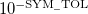are treated as zero.

TYPE:
 INTEGER

DEFAULT:
 5 corresponding to.

OPTIONS:
 User defined.

RECOMMENDATION:
 Use the default unless the molecule has high symmetry which is not being correctly identified. Note that relaxing this tolerance too much may introduce errors into the calculation.